Question

Find dy/dx of the next relations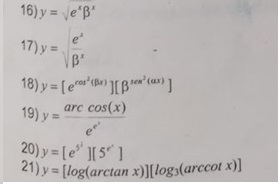I have used Product rule of Differentiation to find out required answer.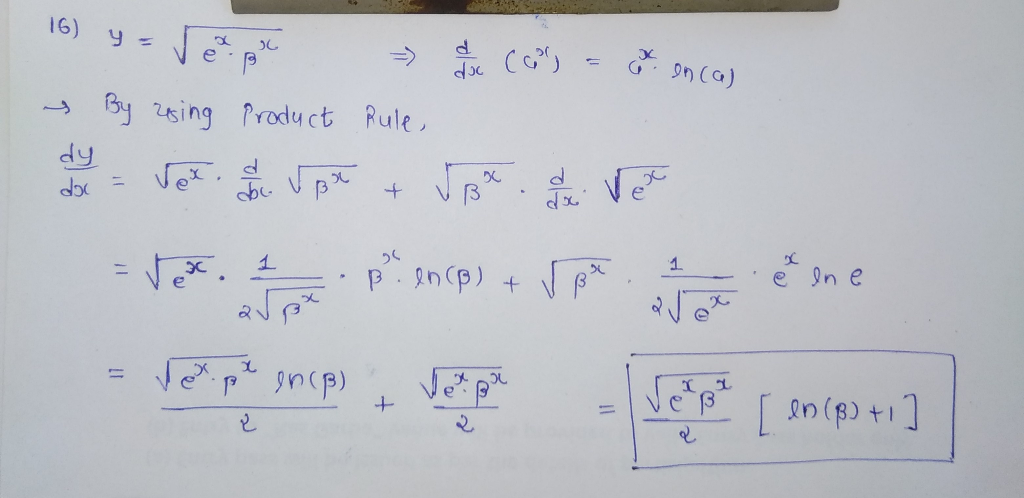Please be informed that I m supposed to do only one question. So, it's my humble request please don't give negative rating because of that reason !!

#### Earn Coins

Coins can be redeemed for fabulous gifts.

Similar Homework Help Questions
• ### Find dy/dx of the next relations      Sol: y ylx 1 1-Cx C 2) 1+cx y' (1+cx1-cx a+bx ab 3) y= In Va-bx y's a2-b&#...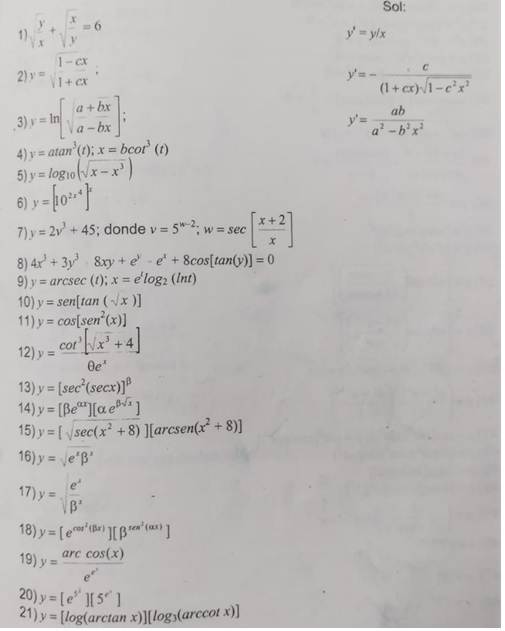Find dy/dx of the next relations      Sol: y ylx 1 1-Cx C 2) 1+cx y' (1+cx1-cx a+bx ab 3) y= In Va-bx y's a2-b'x 4) y= atan (t); x = bcor (t) 6) x+2 7) y = 2v+ 45; donde v 52., W sec X 8)4x+3 8xy+e -e+ 8cos[tan(y)] = 0 arcsec (); x = elog2 (Int) 9) y 10) y sen[tan(x )] 11) y = cos[sen' (x)] cot + 4 12) y 13) y = [sec'(secx))P 14) y [Beae...

• ### (a) [1[*(2x*y + 4y2) dy dx (b) ["" ["(y cos(x) + 6) dy dx cos [**(buye*...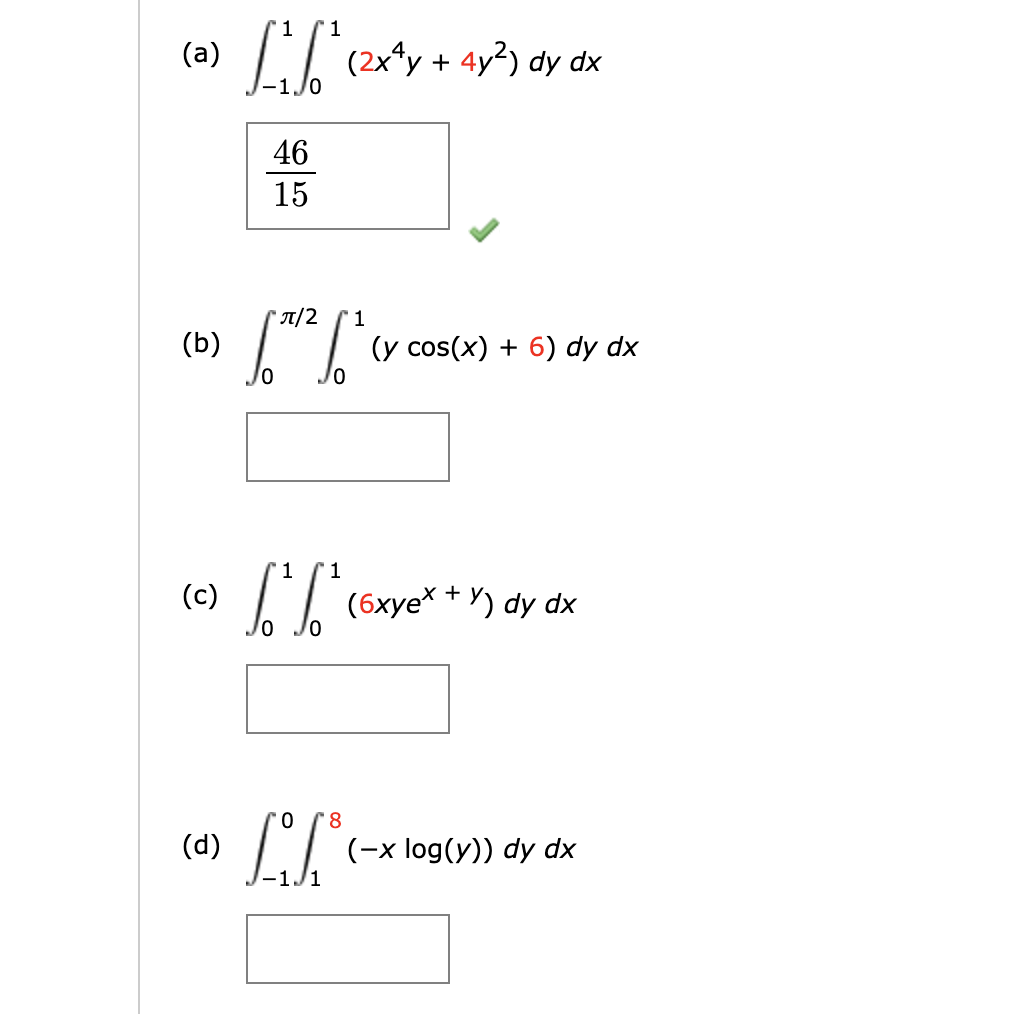(a) [1[*(2x*y + 4y2) dy dx (b) ["" ["(y cos(x) + 6) dy dx cos [**(buye* * *) ay ox (a) LiS.*r-* log(4) dy dx (-x log(y)) dy dx -Il

• ### dy Find dx for the following function. y = 9 sin x + 5 cos x...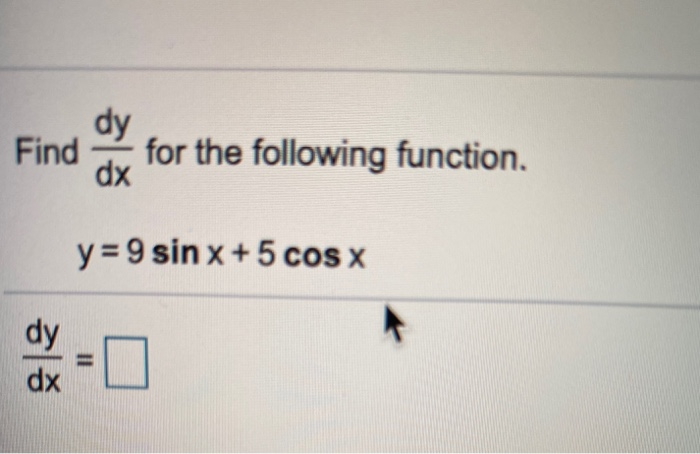dy Find dx for the following function. y = 9 sin x + 5 cos x dy dx 1

• ### Find a solution 6. (e* sin y + tan y)dx + (e* cos y + x(sec...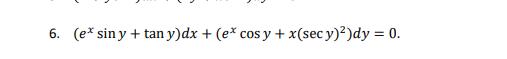Find a solution 6. (e* sin y + tan y)dx + (e* cos y + x(sec y)2)dy = 0.

• ### find a solution 6. (e* sin y + tan y)dx + (e* cos y + x(sec...find a solution 6. (e* sin y + tan y)dx + (e* cos y + x(sec y)2)dy = 0.

• ### Find dy/dx of the next relations 12)y - cor +4 13) y [sec (secx)] 14) y [Be"ae ] 15) y = [ vsec(x2 +8) arcsen(x + 8...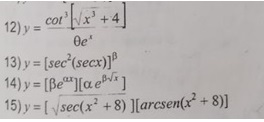Find dy/dx of the next relations 12)y - cor +4 13) y [sec (secx)] 14) y [Be"ae ] 15) y = [ vsec(x2 +8) arcsen(x + 8)) 12)y - cor +4 13) y [sec (secx)] 14) y [Be"ae ] 15) y = [ vsec(x2 +8) arcsen(x + 8))

• ### 2. Solve the DE: (3x²y + cos x) dx +(x +e") dy = 0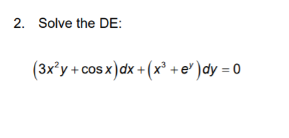2. Solve the DE: (3x²y + cos x) dx +(x +e") dy = 0

• ### 4. (a Let (sin( x cos( ) dr + (x cos(x + y) - 2) dy....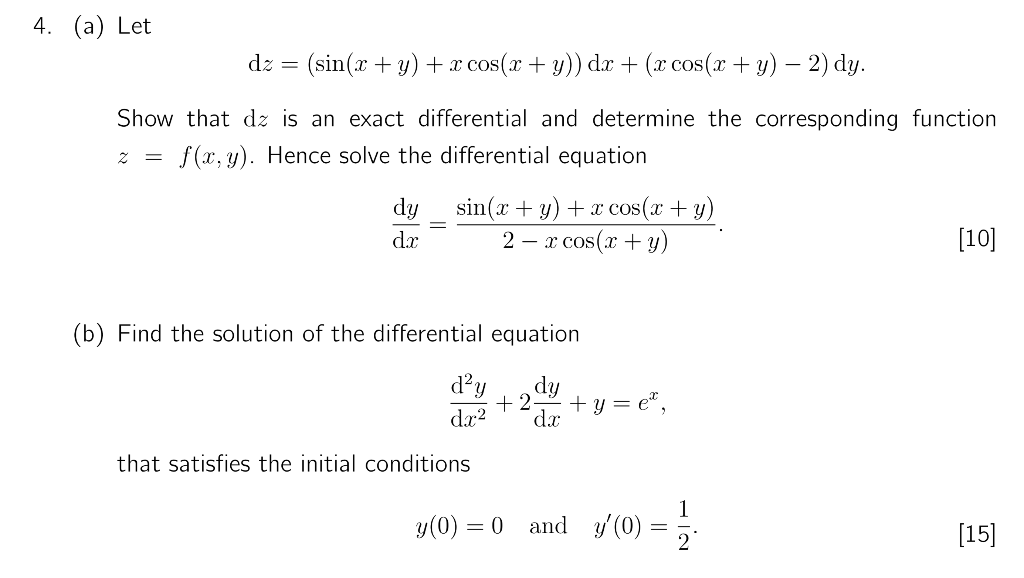4. (a Let (sin( x cos( ) dr + (x cos(x + y) - 2) dy. dz= Show that dz is an exact differential and determine the corresponding function f(x,y) Hence solve the differential equation = z sin( Cos( y) 2 x cos( y) dy 10] (b) Find the solution of the differential equation d2y dy 2 y e dx dæ2 initial conditions th that satisfi 1 (0)  and y(0) 0 4. (a Let (sin( x cos( ) dr...

• ### 19-20-21 19. [5pts.Each] Find the derivative of the functions: sin x a. y = b. g(x)=e*...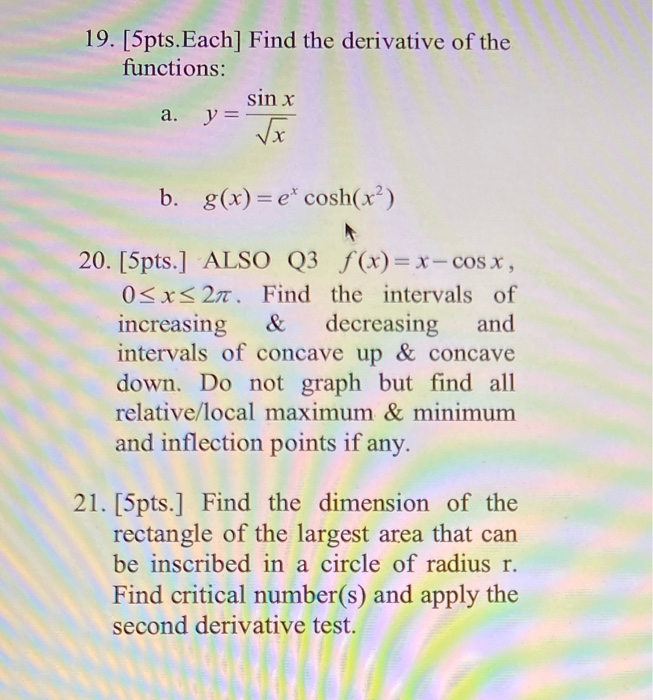19-20-21 19. [5pts.Each] Find the derivative of the functions: sin x a. y = b. g(x)=e* cosh(x²) 20. [5pts.] ALSO Q3 f(x)= x - cos x, 0<x< 277. Find the intervals of increasing & decreasing and intervals of concave up & concave down. Do not graph but find all relative/local maximum & minimum and inflection points if any. 21. [5pts.] Find the dimension of the rectangle of the largest area that can be inscribed in a circle of radius r....

• ### Find the solution to the initial value problem: dy dy/dx=x^ 2√1 + x^3/1+cos y y(0)=2 the...

Find the solution to the initial value problem: dy dy/dx=x^ 2√1 + x^3/1+cos y y(0)=2 the 1+x^3 is all in square root.